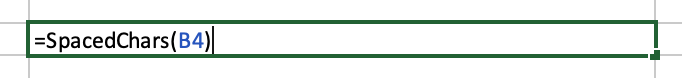# Split Characters in an Excel File

Question:

I have a column in Excel, it has text like:

``````arrow-keys
B
b-
bank
baseball
baseball-bat
baseball-glove
Android-send-text-message
``````

Desired output:

``````a r r o w - k e y s
a v o c a d o
B
b -
b a d g e r
b a g e l
b a n k
b a s e b a l l - b a s e
b a s e b a l l - b a t
b a s e b a l l - g l o v e
A n d r o i d - s e n d - t e x t - m e s s a g e``````

this works

As a footnote, very recent builds of macOS MS Excel (those which support `LAMBDA` expressions in cell formulae) allow you to define and name a custom function which does this:

The help system in these builds shows you how to:

• test a formula,
• wrap it in `=LAMBDA(valueName, outputValueFormula)`,
• and then give this custom function a name under `Formulas > Define Name`.

for example, if we have defined a custom function which returns an Array version of an input string, and given it the name `CHARS` under `Formulas > Define Name`:

``````=LAMBDA(string, MID(string,ROW(INDIRECT("1:"&LEN(string))),1))
``````

we can then use `CHARS` inside the definition of another custom function – let's call it `SPACEDCHARS`:

``````=LAMBDA(S, CONCAT(LAMBDA(x, x & " ")(CHARS(S))))
``````

and then use `SPACEDCHARS` as in the screenshot above.

e.g.

``````=SPACEDCHARS(B4)
``````

and, FWIW, another definition of `SPACEDCHARS` (combining our user-defined `CHARS` with the built-in `TEXTJOIN`), might be:

``````=LAMBDA(s, TEXTJOIN(" ",TRUE,CHARS(s)))
``````

To summarize:

``````CHARS
=LAMBDA(s, MID(s, ROW(INDIRECT("1:"&LEN(s))), 1))

SPACEDCHARS
=LAMBDA(s, TEXTJOIN(" ", TRUE, CHARS(s)))
``````

Under `Formulas > Define Name`:

The part that I might use Keyboard Maestro for is:

• The storage of a collection of such definitions
• and the export of some sub-set of them into a selected Excel workbook.

lambdaExample.xlsx.zip (8.0 KB)

2 Likes

impressive! thanks

A more generally useful user-defined function might, on reflection, be INTERCALATE, which we could specialise with any delimiter (not just a single white space), so given:

``````CHARS
=LAMBDA(string, MID(string, ROW(INDIRECT("1:" & LEN(string))), 1))

INTERCALATE
=LAMBDA(delim, LAMBDA(xs, TEXTJOIN(delim, TRUE, xs)))
``````

we could either write:

or variants like:

and:

1 Like

For regular Excel, found this VBA code that works on OSX:

You can use the following user-defined function to add space between characters or every digits in Excel.

1. Press Alt + F11 keys simultaneously to open the Microsoft Visual Basic for Applications window. (or Go to Developer Tab and select Visual Basic)

2. In the Microsoft Visual Basic for Application window, click Insert > Module. Then copy and paste the following code into the Module window.

``````##### VBA Code: Add Space Between Characters Or Every Digits

Function AddSpace(Str As String) As String
Dim i As Long
For i = 1 To Len(Str)
Next i
End Function
``````
1. Press Alt + Q keys to close the Microsoft Visual Basic for Applications window and get back to the Excel window.

2. Select a blank cell, enter formula =AddSpace(B2) into the Formula Bar, then press the Enter key.

In this case, you can see spaces are added between characters of cell B2.

5.- Note, save the file as a Excel Macro-Enabled Workbook (.xlsm)
and next time you open the file, press Yes when asked "Do you want to enable Macros?"

3 Likes

I am a big fan of VBA, and have used it for decades. If my workflow only needs to automate MS apps, I will often use only VBA. It is very feature rich, and fairly easy to code. One huge help is that you can record a VBA macro using mouse and keyboard to get the basics of macro. Then just edit the VBA macro as needed.

1 Like

and if you wanted a declarative version (avoiding loops), you could write:

``````Function SpacedChars(Str As String) As String
SpacedChars = Join(Split(StrConv(Str, vbUnicode), vbNullChar), " ")
End Function
``````1 LikeOddly enough, ended up with a solution that doesn't involve KM, as the macro took an extremely long time.
It took KM 10 minutes to go through a 4k row file, and sometimes the AppleScript timed out.

That does not sound odd to me, particularly if the KM Macro is in a loop that is doing an Execute AppleScript for every row.

Did you use VBA for your solution?

yes, settled on the last suggestion given by CP, plus a Python script (csvkit) that extracts one column from the Excel file, and now finish the process in about 2-3 seconds.

1 Like